Static and Kinetic Friction

• Last Updated : 05 Jul, 2021

Friction is a force resisting relative motion and it occurs at the interface between the bodies, but also within the bodies, like in case of fluids. The concept of friction coefficient was first formulated by Leonardo da Vinci. The magnitude of the coefficient of friction is determined by the properties of the surfaces, surroundings, surface features, presence of the lubricant, etc.

Laws of Friction

There are five laws of friction and they are:

• The friction of the moving object is proportional and perpendicular to the normal force.

• The friction experienced by the object is dependent on the nature of the surface it is in contact with.

• Friction is independent of the area of contact as long as there is an area of contact.

• Kinetic friction is independent of velocity.

• The coefficient of static friction is greater than the coefficient of kinetic friction.

When we see any object, we can see the smooth surface but when the same object is viewed under a microscope, it can be seen that even the smooth appearing object has rough edges. Tiny hills and grooves can be seen through the microscope, and they are known as irregularities of the surface. So, when one object is moved over the other, these irregularities on the surface get entangled giving rise to friction. More the roughness, more will the irregularities and greater will be the force applied.

Static Friction

There are several theories regarding the causes of static friction, and like most friction-related concepts, each one proves valid under some conditions, but fails under other circumstances. For real-world applications (especially those related to industrial machinery and motion). Control the two most widely-accepted theories behind static friction have to do with the microscopic roughness of surfaces.

Regardless of how “perfectly” a surface is machined, finished, and cleaned, it will inevitably have asperities – essentially “roughness,” consisting of peaks and valleys, much like a mountain range. (Technically the “peaks” are the asperities.) When two surfaces are in contact, it may appear that they have a large, well-defined area of contact, but in reality, contact occurs only at certain places – that is, where the asperities of both surfaces interfere.

The sum of these small areas of contact between the asperities is referred to as the “real” or “effective” area of contact. Because these individual areas of contact are very small, the pressure (pressure = force ÷ area) between the surfaces at these points is very high. This extreme pressure allows adhesion to occur between the surfaces, via a process known as cold welding, which occurs at the molecular level. Before the surfaces can move relative to each other, the bonds that cause this adhesion must be broken.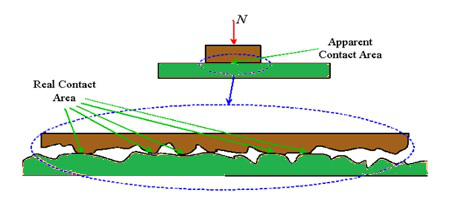In addition, the roughness of the surfaces means that at some locations, the asperities of one surface will settle into the valleys of the other surface – in other words, the surfaces will interlock.

These interlocked areas must be broken or plastically deformed before the surfaces can move. In other words, abrasion must occur. So, in most applications, static friction is caused by both adhesion and abrasion of the contacting surfaces.

Laws Of Static Friction

There are two laws of static friction:

1. First law: The maximum force of static friction is not dependent on the area of contact.
2. Second law: The maximum force of static friction is comparative to the normal force i.e., if the normal force increases, the maximum external force that the object can endure without moving, also increases.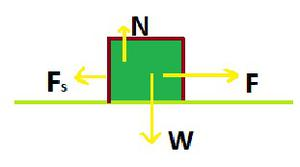Derivation for the formula of static friction

Let us consider a block of weight mg lying on a horizontal surface as shown in the figure. When a body press against a surface, the surface deforms even if it appears to be rigid. The deformed surface pushes the body with a normal force R that is perpendicular to the surface. This is called normal reaction force. It balances mg that is

R = mg

Now let us consider that a force P is applied on the block. Clearly the body remains at rest because some other force F comes into play in the horizontal direction and opposes the applied force P resulting in net force zero on the body. This force F acting along the surface of the body in contact with the surface of the table is called frictional force.

So as long as the body doesn’t move F = P. This means that if we increase P, friction F also increases, remaining equal to P always.

This frictional force which comes into play until the actual motion has started is known as static friction.

Coefficient Of Static Friction

Static Friction is friction which is experienced when an object is placed on a surface. And, Kinetic friction is due to the movement of an object on a surface. Friction is well characterized by the coefficient of friction and is explained as the ratio between the frictional force and the normal force. This helps the object to lie on a surface. The coefficient of static friction is a scalar quantity and denoted as μs.

The formula for the coefficient of static friction is expressed asWhere

μs​ = coefficient of static friction

F = static frictional force

N = normal force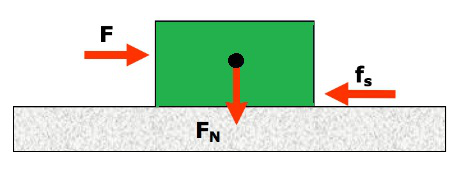Kinetic Friction

Kinetic friction is defined as a force that acts between moving surfaces. A body moving on the surface experiences a force in the opposite direction of its movement. The magnitude of the force will depend on the coefficient of kinetic friction between the two materials.

Friction is easily defined as the force that holds back a sliding object. The kinetic friction is a part of everything and it interferes motion of two or more objects. The force acts in the opposite direction to the way an object wants to slide.

If a car has to stop, we apply brakes and that is exactly where the friction comes into play. While walking, when one wants to suddenly come to a halt, friction is to thank again. But when we have to stop in the middle of a puddle, things get harder since friction is less there and cannot aid one so much.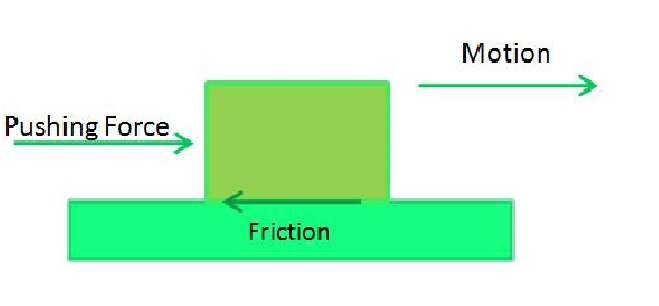Overcoming the static friction between two surfaces essentially removes both the molecular obstacles (cold welding between asperities) and, to some degree, the mechanical obstacles(Interference between the asperities and valleys of the surfaces) to movement. Once movement is initiated, some abrasion continues to occur, but at a much lower level than during static friction and the relative velocity between the surfaces provides insufficient time for additional cold welding to occur (except in the case of extremely low velocity).

With most of the adhesion and abrasion being overcome to induce movement, the resistance to motion between the surfaces is reduced, and the surfaces are now moving under the influence of kinetic friction, which is much lower than static friction.

Laws of Kinetic Friction

There are four laws of kinetic friction:

1. First law: The force of kinetic friction (Fk) is directly proportional to the normal reaction (N) between two surfaces in contact. Where, μk​ = constant called the coefficient of kinetic Friction.
2. Second law: Force of kinetic friction is independent of shape and apparent area of the surfaces in contact.
3. Third law: It depends upon nature and material of surface in contact.
4. Fourth law: It is independent of the velocity of object in contact provided the relative velocity between the object and the surface is not too large.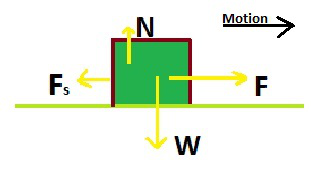Formula of Kinetic Friction

The coefficient of kinetic friction is denoted by the Greek letter “mu” (μ), with a subscript “k”. The force of kinetic friction is μk times the normal force on a body. It is expressed in Newton (N).

The kinetic friction equation can be written as:

Force of kinetic friction = (coefficient of kinetic friction)(normal force)

Fk = μk ​η

Where,

Fk = force of kinetic friction

μk coefficient of kinetic friction

η = normal force (Greek letter “eta”)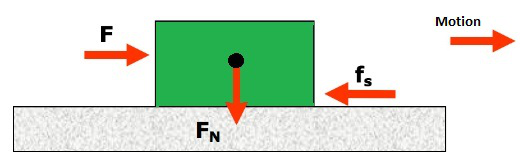Derivation for the Formula of Kinetic Friction

Let us consider a block of weight mg lying on a horizontal surface as shown in the figure. When a body press against a surface, the surface deforms even if it appears to be rigid. The deformed surface pushes the body with a normal force R that is perpendicular to the surface. This is called normal reaction force. It balances mg that is R = mg.

Now let us consider that a force P is applied on the block as shown. Clearly the body remains at rest because some other force F comes into play in the horizontal direction and opposes the applied force P resulting in net force zero on the body. This force F acting along the surface of the body in contact with the surface of the table is called frictional force.

So as long as the body doesn’t move F = P. This means that if we increase P, friction F also increases, remaining equal to P always.

As we increase the applied force slightly beyond limiting friction, the actual motion starts. This does not mean that the friction has disappeared. It only means that the force has overcome the limiting friction. This force of friction at this stage is known as Kinetic friction or Dynamic friction.

The Kinetic friction or dynamic friction is the opposing force that comes into play when on body is actually moving over the surface of another body.

Application of Static and Kinetic Friction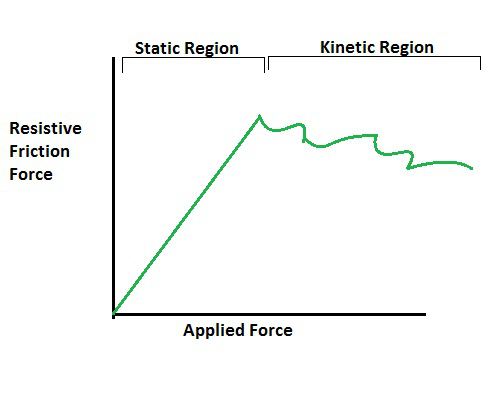Applications of Static Friction

Some real-life examples of static friction are given in the points below:

• Papers on a table-top
• A towel hanging on a rack
• A bookmark in a book
• A car parked on a hill

Applications of Kinetic Friction

Some real-life examples of kinetic friction are given in the points below.

• Friction also plays a huge role in everyday occurrences like while rubbing of two objects takes place. The resulting motion converts into heat and thus resulting in the fire in some instances.
• It is also responsible for wear and tear and that’s why we need oil to lubricate machine parts, as it reduces friction.
• When two objects are rubbed against each other, the frictional force is converted into thermal energy, in few cases giving rise to fire
• Kinetic friction is responsible for the wear and tear of machine parts, hence it is important to lubricate the machine parts with oil.

Sample Problems based on Static and Kinetic Friction

Question 1: A man pushes large cardboard of mass 75.0 kg box across the floor.

Solution:

The coefficient of kinetic friction is μk = 0.520​

The worker exerts a force of 400.0 N forward.

What is the magnitude of the force of friction?

Answer: On a flat surface, the normal force of an object can be found out by the formula

η = mg

By substituting the value of η in the equation Fk = μk​ η , we get

Fk= (0.520) (75.0kg) (9.80m/s2) = 382.2N

Question 2: In the above question, calculate the net force moving the box?

Solution:

The net force acting on a body is the sum of all the forces acting on the body.

In this case, the forces acting on the body are the force exerted by the man and the kinetic friction acting in the opposite direction.

If the forward motion is considered positive, then the net force is calculated as follows:

Fnet = Fworker – Fk

Substituting the values in the above equation, we get

Fnet = 400 N – 382.2 N = 17.8 N

Question 3: Why does rolling motion experience friction?

In theory, a ball makes point contact with the surface.

But in reality, the ball (and/or the surface) deforms due to the load, and the contact area becomes elliptical.

In theory, rolling surfaces, such as those found in most rotary and linear bearings (except plain bearings), should not encounter friction forces.

But in real-world applications, three factors cause friction in rolling surfaces:

1. Micro-slip between the surfaces (the surfaces slide relative to one another)

2. Inelastic properties (i.e. deformation) of the materials

3. Roughness of the surfaces

Question 4:An object having a mass of 10 kg is placed on a smooth surface. Static friction between these two surfaces is given as the 15 N. Find the coefficient of static friction?

Solution:

Given

m = 10 kg

F = 30 N

μs = ?

We know that,

Normal force, N = mg

So, N = 10× 9.81 = 98.1 N

The formula for coefficient of static friction is,

μs = F/N

μs = 30/98.1

μs = 0.305

Question 5: The normal force and the static frictional force of an object are 50 N and 80 N respectively. Find the coefficient of static friction?

Solution:

Given

N = 50 N

F = 80 N and μs = ?

The formula for coefficient of static friction is

μs = F/N

μs = 80/50

μs = 1.6

Question 6: What is the relation between static and kinetic friction?

The Force of Static Friction keeps a stationary object at rest. Once the Force of Static Friction is overcome, the Force of Kinetic Friction is what slows down a moving object.

Question 7: A fridge weighs 1619 N and the static friction coefficient is 0.50. What is the least force used to move the fridge?

Solution:

Given data:

Weight of the fridge, W=1619 N

W=1619 N

Coefficient of static friction, μs = 0.50

The minimum force needed to move the fridge can be given as,

F = μs

F = 0.50 × 1619

F = 809.50 N.

My Personal Notes arrow_drop_up